# Reflection at curved surfaces

### Guest Account

Attempt Form Two Physics Questions
Guest Account

# Reflection at curved surfaces

## Concave and convex mirrors

-They are also known as spherical mirrors and are formed when a spherical glass is silvered.
-If the inside is silvered a convex or diverging is formed while a concave or converging mirror is formed when the outside is silvered.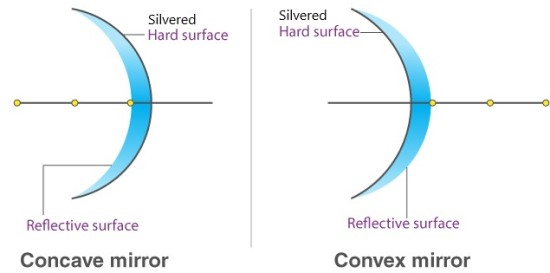#### Parts of a spherical mirror

1. Centre of curvature (C) – this is the centre of the sphere of which the mirror is part of. The centre itself is called the pole (P).
2. Principal axis – this is the line joining the centre of curvature (C) to the pole (P).
3. Principal focus (F) – is a point on the principal axis through which a ray is reflected when it hits a concave mirror. In a convex mirror the ray is reflected and appears to originate from the point. F is virtual for a convex mirror while it is real for a concave mirror.
4. Radius of curvature (r) - this is the distance from the pole to the centre of curvature. The distance from the pole to the principal focus is called the focal length (f).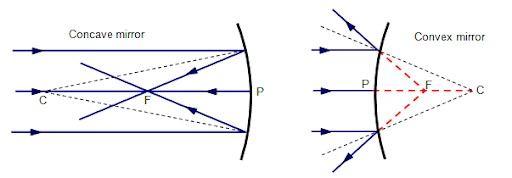#### Parabolic mirrors

- They produce a wide parallel beam or converge a large beam of light to a point.
- They are widely used in making car headlights or in spotlights.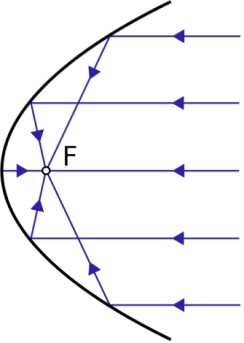## Images formed by spherical mirrors

#### Location of images using ray diagrams

- When drawing ray diagrams the following symbols are used to represent the mirrors.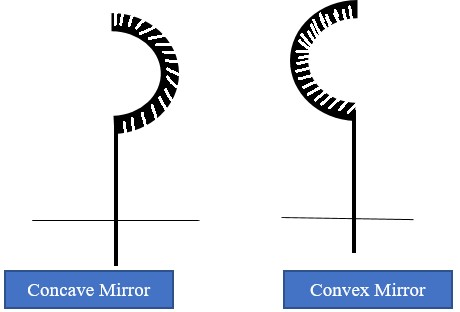The image is located by drawing any two of the following rays:
1. A ray parallel to the principal axis which is reflected through the principal focus.
2. A ray through the centre of curvature which is reflected along its own path since it hits the mirror normally.
3. A ray through the principal focus which is reflected parallel to the principal axis.
- Virtual images are formed when rays diverge and as such the rays are extended backwards using dotted line till they meet.
- A real image is formed by intersection of real rays.

#### Concave mirror

a) Object at infinity: image is formed at F. It is real, inverted and diminished.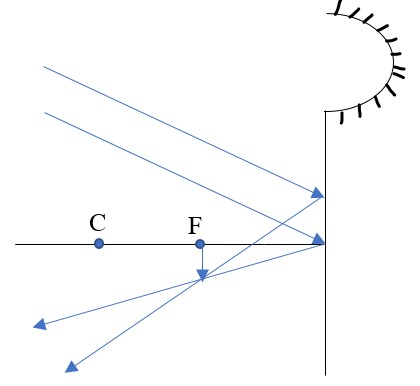b) Object at C: image formed at C. It is real, inverted and the same size as the object.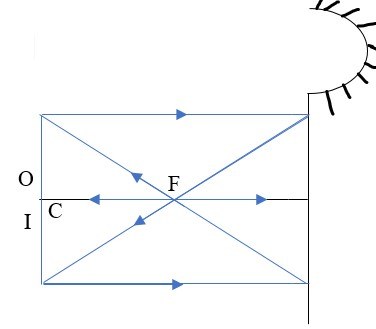c) Object behind C: image is formed between C and F. It is real, inverted and diminished.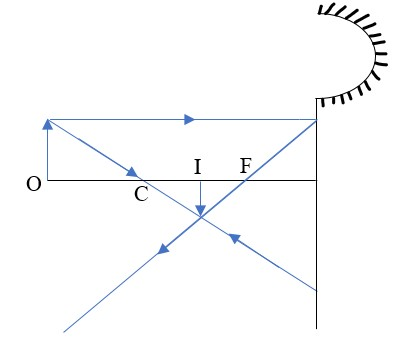d) Object between F and C: Image is formed behind C. It is real, inverted and magnified.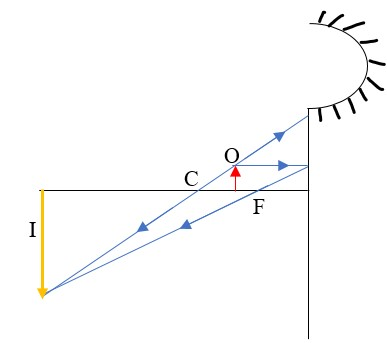e) Object at F: Image formed is at infinity.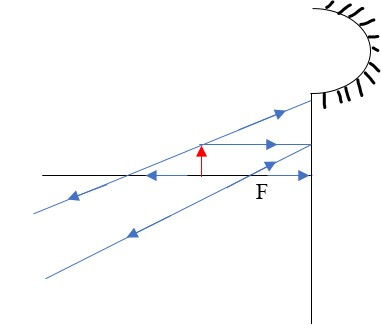f) Object between F and P: Image is formed behind the mirror. It is virtual, erect and magnified.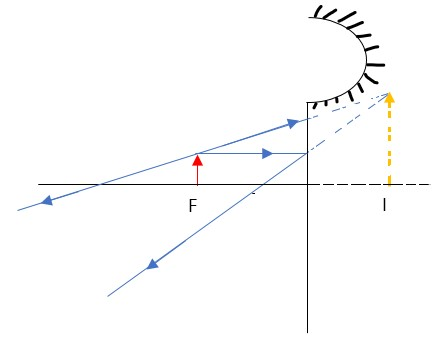#### Convex mirror

a) Image is always formed behind the mirror. It is virtual, erect and always diminished.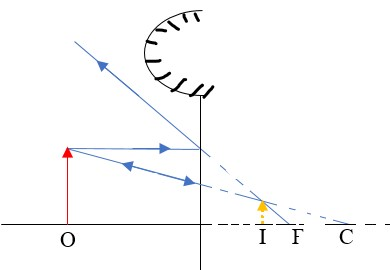Applications of curved reflectors
a) They are used in satellite dishes.
b) They are used in making shaving mirrors.
c) They are used in telescopes.
d) They are used in driving mirrors.

## Magnification

- Magnification is the ratio of the image size to the object size.
- Magnification (M) = height of the image / height of the object.
- When the ratio is greater than one we say the image is magnified and when less than one we say it is diminished.
- Also magnification = image distance from the mirror / object distance from the mirror.

Example 1
1. Determine the size, position and nature of the image of an object 5.0 cm tall, placed on the principal axis of a concave mirror of focal length 15 cm, at a distance 35 cm from the mirror.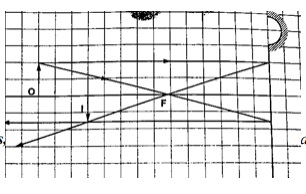Solution
Let 1 cm represent 5 cm. Then the focal length is 3 cm.
Object distance = 7 cm, object height = 1 cm.
From the scale drawing,
Image position = 5.4 cm × 5 = 27 cm in front of the mirror.
Image size = 0.75 cm × 5 = 3.75 cm.
Image is real and inverted.

Example 2
2. A vertical object 5 cm high is placed 10 cm in front of a convex mirror of focal length 15 cm. find the position, size and nature of image formed. Determine the magnification of the image.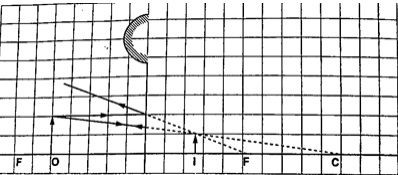Solution
Let 1 cm represent 5 cm, then the focal length = 3 cm, object size = 1 cm
Object distance = 2 cm.
From the scale drawing,
Image position = 1.2 cm × 5 = 6.0 cm behind the mirror.
Image size = 0.6 cm × 5 = 3.0 cm.
The image is virtual and erect.
Magnification = image distance / object distance. Hence 6 /10 = 0.6 (diminished).